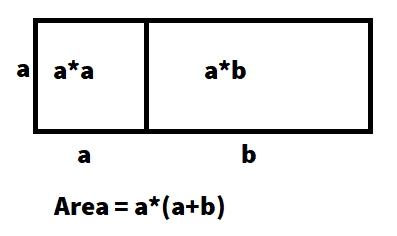# Manipulating exponents: not sure how this example is worked out

geologist
Member has been advised to use the template and show some effort.
Summary: An example problem on Brilliant.org leaves out a step in solving 2^3 + 6^3

I've spend a couple days trying to understand how the second step of solving the problem 23 + 63, which is 23 + 63 = 23 + (23 * 33) = 23(1 + 33).

It is not clear how this problem gets to 23(1 + 33). I can see that somehow you have to factor one of the 23, but I cannot visualize how that is done. What concept should I review to understand this problem?

Homework Helper
Gold Member
Just a quick look at your post #1,
...
DISTRIBUTIVE PROPERTY

•jedishrfu
Homework Helper
Gold Member
2^3+6^3
2^3+(2*3)^3
2^3+(2^3*3^3)
2^3+(2^3)(3^3)
(2^3)(1+3^3), factorizable because of the Distributive Property

•jedishrfu
Mentor
2022 Award
I cannot visualize how that is done.
It is how an area can be calculated:•jedishrfu
Homework Helper
Gold Member
fresh_42, I have occasionally used that kind of visualizing, too. When trying to factorize an expression, one must actually see the factors IN the expression.

geologist
2^3+6^3
2^3+(2*3)^3
2^3+(2^3*3^3)
2^3+(2^3)(3^3)
(2^3)(1+3^3), factorizable because of the Distributive Property

I understand how a(b-c) = ab - ac and how (5x+2)(5x+2) = 25x^2 + 20x + 4, but I'm struggling to understand what is "a" in the above problem. I'll have to digest this information and read more examples on the distributive property.

Mentor
2022 Award
I understand how a(b-c) = ab - ac and how (5x+2)(5x+2) = 25x^2 + 20x + 4, but I'm struggling to understand what is "a" in the above problem. I'll have to digest this information and read more examples on the distributive property.
The expression you have is ##a+(a\cdot b)##. Now we first write it as ##a\cdot 1 + a\cdot b## so we have a common factor ##a## in both terms, which allows us to use the distributive law backwards:
$$a\cdot 1 + a\cdot b = a\cdot (1+b)$$

geologist
The expression you have is ##a+(a\cdot b)##. Now we first write it as ##a\cdot 1 + a\cdot b## so we have a common factor ##a## in both terms, which allows us to use the distributive law backwards:
$$a\cdot 1 + a\cdot b = a\cdot (1+b)$$
That make sense. I understand what’s going on now. The step 2^3+(2^3*3^3) is unnecessary. My confusion with 2^3(1+3^3) can be understood better looking at the originally stated problem 2^3 + 3^3. Now it’s easy to see how 2^3 factors out.

Homework Helper